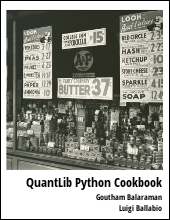This handbook gives a concise introduction to option models. Part I gives a basic introduction to principles behind option model pricing

This is a series of articles pertaining to option model pricing. Here I will explain the various concepts such as risk-neutral valuation, Black-Scholes formula, and binomial tree option pricing models.

## Introduction to Option Models

The bedrock of options pricing is the risk-neutral valuation principle, which relates the expected value of a ﬁnancial product at a future time to its current price. This is consistent with no-arbitrage hypothesis. Vanilla options have a theoretical price given by the Black-Scholes formula. In this article we will briefly touch upon these two concepts.

### Risk-Neutral Valuation

An important general principle in options pricing is the risk-neutral valuation. According to this principle, the expected return from a stock at time $T$, $E(S_T)$, is the risk free value of the current stock price:

$$E(S_T) = S_0 e^{rT}$$

The continuous compounding risk-free rate is $r$ and the current stock price is $S_0$. The principal of risk-neutral valuation can be used to create a binomial model for price movement, and subsequently a method to value options.

### Black-Scholes Formula

Value of a vanilla European call option, struck at $K$ with time $T$ to maturity, can be derived using the above mentioned risk-neutral valuation principle. The payoﬀ of the call at maturity on an underlying with price $V$ (at maturity) is $max(V − K, 0)$. The expected value of this payoﬀ can be found, assuming a geometric brownian motion price movement for the underlying as,

\begin{equation} E[max(V-K,0)] = \int_K^\infty (V-K)g(V)dV \end{equation}

where $g(V)$ is the probability density function of $V$ such that $log(V)$ is normally distributed with standard deviation $w$. Thus the current value of the call is

$$C = e^{-rT} E[max(V-K,0)]$$

which after a lengthy calculation comes out to be

\begin{equation} \label{eq:bs} C = S_0 N(d_1) - K e^{-rT} N(d_2) \end{equation}

where $S_0$ is the current price of the underlying, the risk free rate $r$, the volatility of the underlying is $\sigma$,

$$d_1 = \frac{ \ln(S_0/K) + (r+\sigma^2/2)T } { \sigma \sqrt{T} }$$

$$d_2 = \frac{ \ln(S_0/K) + (r-\sigma^2/2)T } { \sigma \sqrt{T} } = d_1 - \sigma\sqrt{T}$$

and $N(x)$ is the cumulative distribution function. The put-call parity relates the price of the put and call prices, and is given as:

$$C - P = S_0 - e^{-rT}K$$

Put-Call parity and Eq. $\ref{eq:bs}$ can be used to arrive at put prices.

For the case where the underlying has a continuous payout (dividend), the Black-Scholes formula can be extended to yield

$$C = S_0 e^{-qT} N(d_1^\prime) - K e^{-rT} N(d_2^\prime)$$

where

$$d_1^\prime = \frac{ \ln(S_0/K) + (r - q + \sigma^2/2)T} {\sigma\sqrt{T}}$$

$$d_2^\prime = d_1^\prime - \sigma\sqrt{T}$$

### Conclusion

In this article I explained the fundamentals of option model pricing. We looked at the risk neutral valuation, and how it can be used to derive the Black-Scholes model.

finance   option models   binomial tree

Related PostI am Goutham Balaraman, and I explore topics in quantitative finance, programming, and data science. You can follow me @gsbalaraman.

Checkout my bookUpdated posts from this blog and transcripts of Luigi's screencasts on YouTube is compiled into QuantLib Python Cookbook .